# GEOMETRIC SEQUENCES Geometric Sequence A geometric sequence is

• Slides: 15GEOMETRIC SEQUENCES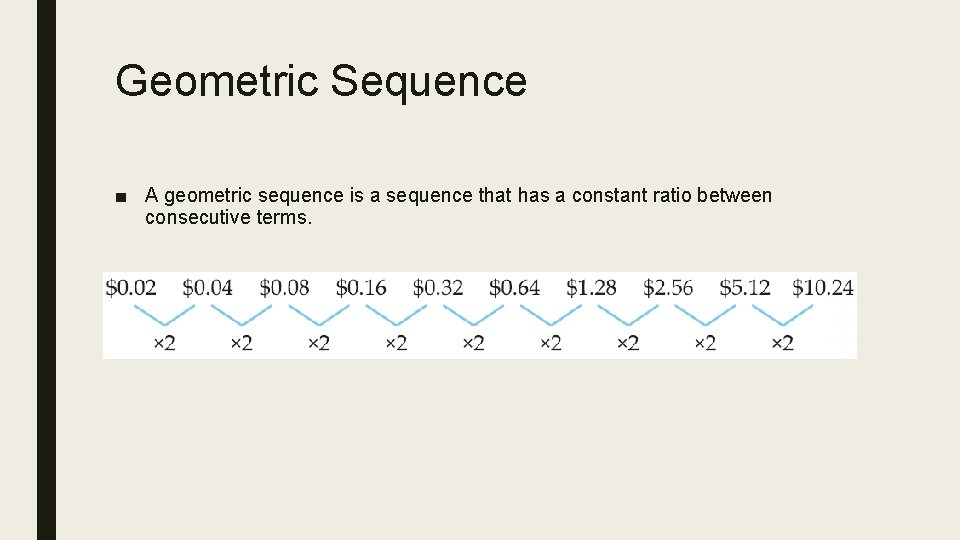Geometric Sequence ■ A geometric sequence is a sequence that has a constant ratio between consecutive terms.Geometric Sequence ■ A geometric sequence has a multiplicative relationship (common ratio) between terms. ■ Terms multiply or divide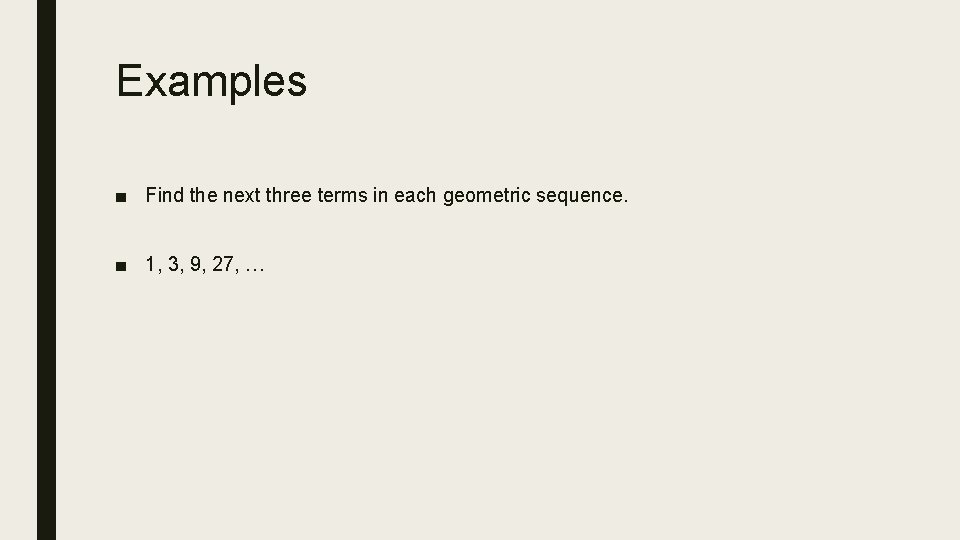Examples ■ Find the next three terms in each geometric sequence. ■ 1, 3, 9, 27, …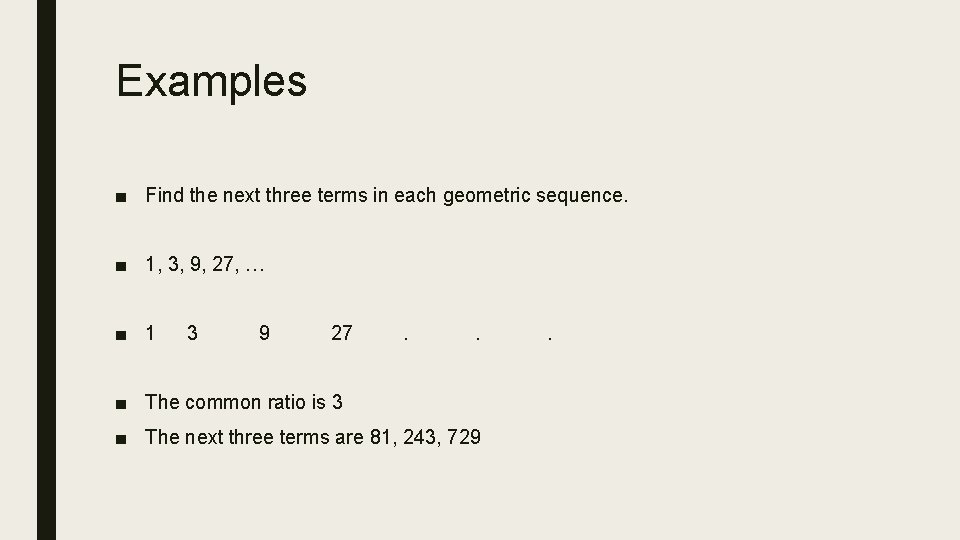Examples ■ Find the next three terms in each geometric sequence. ■ 1, 3, 9, 27, … ■ 1 3 9 27 . . ■ The common ratio is 3 ■ The next three terms are 81, 243, 729 .Examples ■Examples ■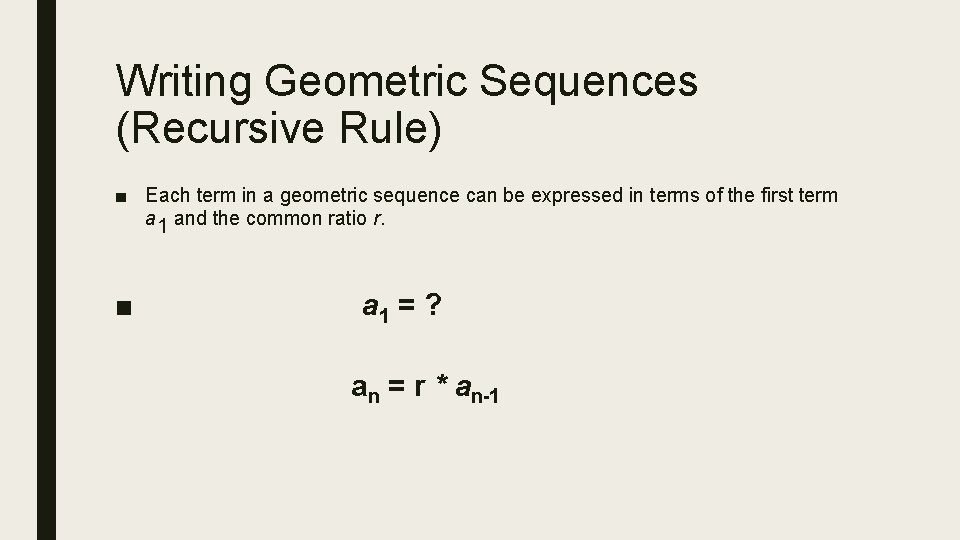Writing Geometric Sequences (Recursive Rule) ■ Each term in a geometric sequence can be expressed in terms of the first term a 1 and the common ratio r. ■ a 1 = ? an = r * an-1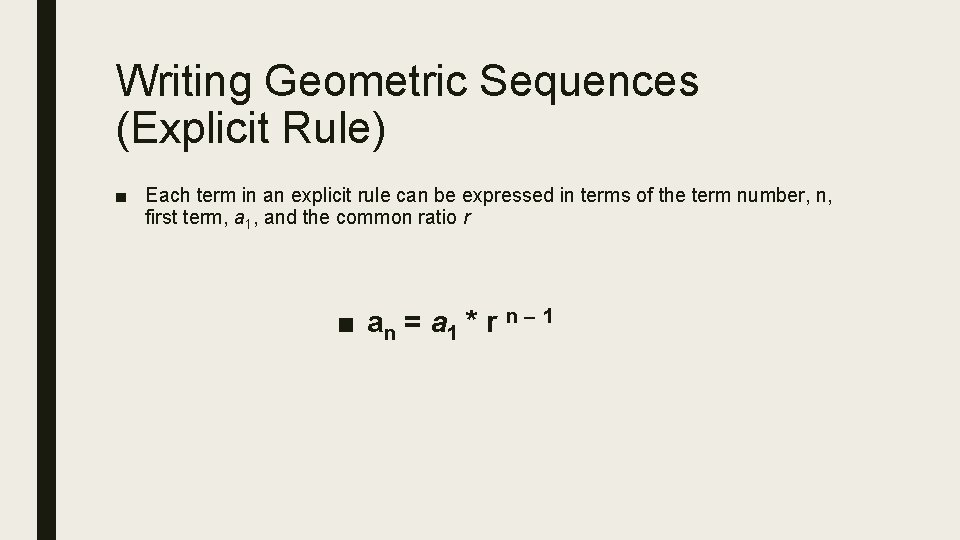Writing Geometric Sequences (Explicit Rule) ■ Each term in an explicit rule can be expressed in terms of the term number, n, first term, a 1, and the common ratio r ■ an = a 1 * r n – 1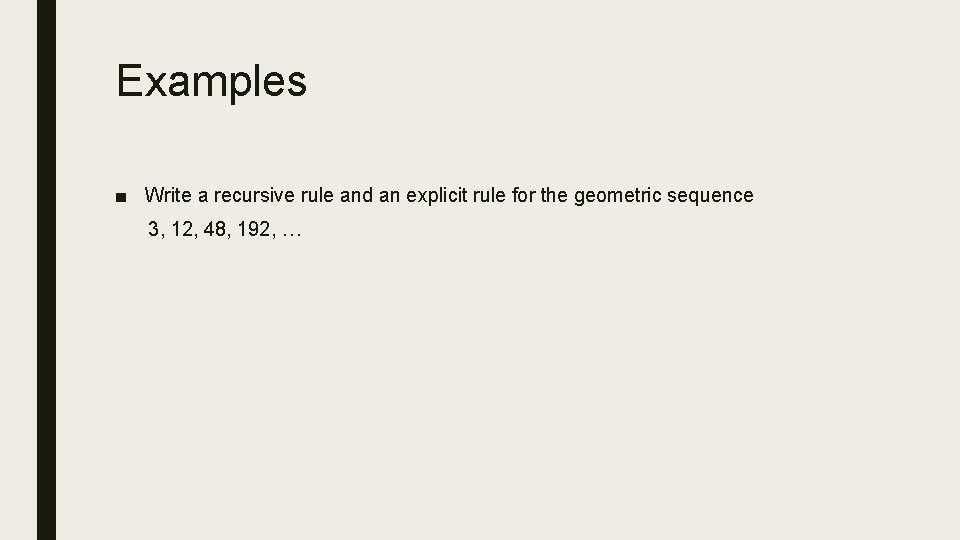Examples ■ Write a recursive rule and an explicit rule for the geometric sequence 3, 12, 48, 192, …Examples Write a recursive rule and an explicit rule for the geometric sequence 3, 12, 48, 192, … Recursive Rule Explicit Rule ■ a 1 = 3 ■ an = a 1 * rn-1 ■ an = 4 an-1 ■ an = 3 * 4 n-1Examples ■ Find the eighth term of a geometric sequence for which a 1 = -3 and r = -2.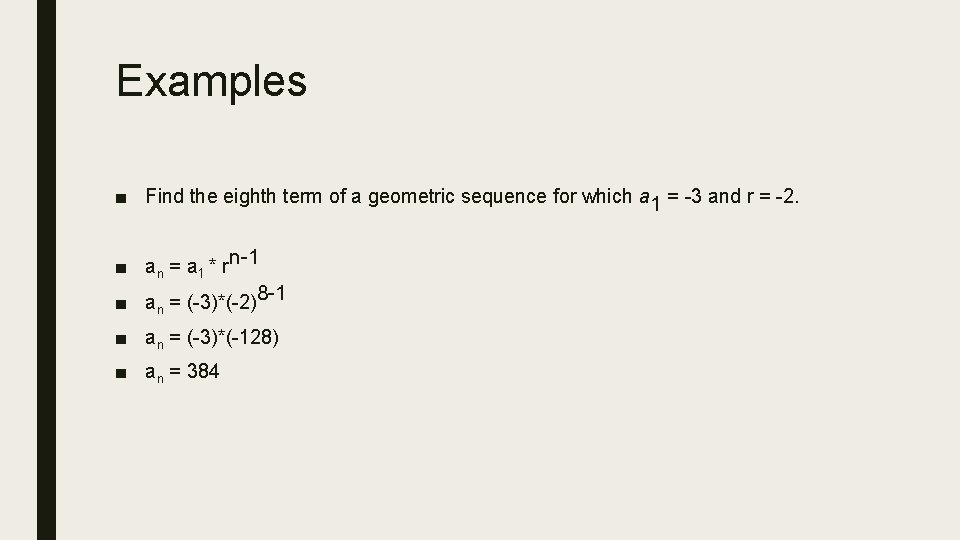Examples ■ Find the eighth term of a geometric sequence for which a 1 = -3 and r = -2. ■ an = a 1 * rn-1 ■ a = (-3)*(-2)8 -1 n ■ an = (-3)*(-128) ■ an = 384Examples ■ Find the tenth term of a geometric sequence for which a 4 = 108 and r = 3.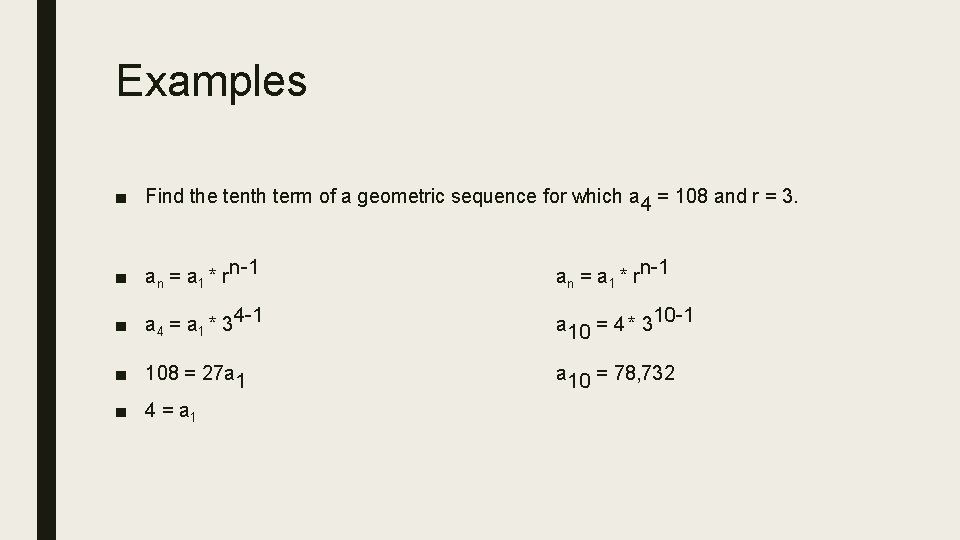Examples ■ Find the tenth term of a geometric sequence for which a 4 = 108 and r = 3. ■ an = a 1 * rn-1 ■ a 4 = a 1 * 34 -1 a 10 = 4 * 310 -1 ■ 108 = 27 a 1 a 10 = 78, 732 ■ 4 = a 1